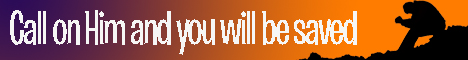Note:  Do not rely on this information. It is very old.

# Extension

Extension means a drawing out. Geometrically, the extension of a substance is regarded as a strain and the cause is called stress. Such may be produced by the direct application of mechanical force, and this expenditure of energy is measured by the product of the force and the extension produced. Thus in the case of mere elongation or extension in one direction only, the product of force and the change of length measures the work done and, therefore, the energy expended. Similarly for extension in two dimensions, the force per unit length multiplied by the change in area gives the work done; and for extension in three dimensions the force per unit area multiplied by the change in volume measures the energy expended. In the case of expansion due to heating we are thus able to measure the heat required to effect this expansion, and can conclude that unless the substance is itself changed the heat energy given to it is measured in the above way.